IF IT'S EDUCATION, IT'S ITS
Pathways To Learning... Since 2005
Hong Kong Registered School 566985

# D - d'Alembert's test to dyne - Mathematics Dictionary

A   B   C   D   E   F   G   H   I   J   K   L   M   N   O   P   Q   R   S   T   U   V   W   X   Y   Z

d'Alembert's test: Also known simply as the ratio test. Attributed to Jean-Baptiste le Rond d'Alembert, it is a criterion for the convergence of a series, which states that a series is convergent if the limit of the ratio between consecutive terms is below 1, divergent if the ratio tends to a number greater than 1 and indeterminate (as far as the test is concerned) if the limit of the ratio is exactly 1.

d' Alembert's theorem: 1. A theorem in geometry regarding a set of 3 circles.

2. Another class="d-title" name for the fundamental theorem of algebra.

damped harmonic motion: The harmonic motion with additional factors which rduces the amplitude of the oscillations. (e.g. friction or air resistance). Just as simple harmonic motions, damped harmonic motions can be described by a second order differential equation: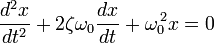where ω0 is called the undamped angular frequency of the oscillations and ζ is a constant known as the damping ratio.

data: Quantitative or qualitative results of observations and measurements in statistics. The word data is the plural of the singular form datum.

day: The class="d-title" name for a number of related units of time all based around the period of the Earth's rotation about its own axis.

deca-: An SI prefix that means ten or ten-fold.

decade: The equivalent power of 10 in the ratio of 2 numbers.

decagon: A polygon of ten sides.

deceleration: A reduction in speed, or equivalently negative acceleration in cases where absolute velocity is considered.

deci-: An SI prefix that means one tenth.

decibel: A logarithmic unit for the ratio of two quantities, or a quantity against a reference quantity. It is one-tenth of a bel, but decibels are much more commonly used. A bel (or more commonly 10 decibels) represents a factor of 10 between 2 quantities.

decile: A value under which a whole number of tenths of data fall.

decimal: A number expressed in a particular positional numbering system of base 10. For example, the number 0.537 is a decimal representation of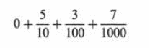decimal fraction: A fraction whose denominators are all powers of ten, as shown under the entry of decimal above..

decimal notation: The graphical representational aspect of the decimal number system.

decimal place: The positions towards the right of the decimal point (indicating quantites less than whole) within the decimal number system.

decimal point: A dot used to separate the integral part of a number from the fractional part in the decimal number system. The point is either centred (as in 0·5) or, now more commonly, placed on the line (0.5), as in this Dictionary. In many European countries a comma is used (0,5).

decimal system: A positional number system of base 10 or the concept of representing numbers using base 10 (independent of symbolic representations). Commonly refers to the base-10 system in widespread use.

decision problem: A problem whose construction only accepts a "yes or no" answer which is dependent entirely on only the parameters of the problem. (Although the answer may not be actually known, decidable or known to be decidable.)

decision theory: A branch of mathematics which deals with making decisions by identifying the relevant parameters, which parameters are known, what the known values are for these parameters and how to use such parameters to achieve an optimal decision by certain standards as well as how such standards are defined, assessed, affect the decision making process and any other aspects concerned.

decision tree: A type of tree diagram used to display information relevant to and support the process of decision making by using probabilities and expected values amongst other mathematical/statistical concepts.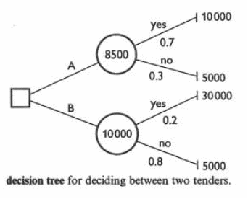decomposition: The process of splitting a mathematical object into two or more objects of the same type, such as fractions, functions or matrices.

decreasing function: A function where x < y implies and is implied by f(x) > f(y). Depending on the author, the second inequality may be ≥ so that a decreasing function is allowed to "level" as well as decrease. In which case, most authors are likely to call the first type strictly decreasing functions. Note that monotonic(-ally) decreasing functions do not mean that the inquality signs are strict but that the decreass is uninterrupted (without exceptions).

decreasing sequence: A sequence a1 a2 a3an such that an >an+1. Similar to the definition of decreasing functions, some author allow anan+1 and refer to the first type as strictly decreasing sequences.

deduction: A method of inference which attempts to "justify" conclusions by applying rules of inference on the premises of the argument and other such conclusions.

deficient number A number which is greater than the sum of its proper divisors, also known as a defective number. As opposed to a perfect number, whose sum of divisors equal itself.

definite integral: An operation on functions which approximates the sum of the values (of the function) weighted by the length (or measure) of the intervals for which the function takes that value. A real valued function ƒ being evaluated (integrated) over the closed interval [ab] is written aswhich can informally be thought of as finding the exact area between the curve and the x-axis between the vertical lines x = a and x = b.

deformation: The effect of a transformation where shapes before and after the transformation are not mathematically similar.

degree: 1. A unit of angle, commonly representing one-ninetieth of a right angle. Although systems where one degree (a different unit) representing one hundredth of a right angle exists.

2. A unit of temperature, commonly either one one-hundredth (Celsius or Kelvin) or one one-hundred-and-eightieth of the temperature difference between the freezing and boiling point of water. (In certain conditions such as standard atmospheric pressure etc.)

3. The index of a variable in a mathematical term.

4. The highest index of a variable in any mathematical terms in a polynomial of one variable.

5. The highest sum of indices of any mathematical terms in a polynomial of more than one variable.

degree measure: A measureent in plane angles in degrees.

degrees of freedom: A number of related concepts in physics, mechanics, engineering and statistics regarding the independence/interdependence of parameters. Informally, any parameters/variables whose value can occur or be set independently of the values of other parameters/variables count as one degree of freedom towards the (total) number of degrees of freedom of the whole system.

del: A unary partial differential operator which is shorthand for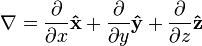which is only concretely defined by its relationship with and effects on functions.

deleted neighborhood: The neighbourhood of a point P - the set of all points within a certain fixed radius of the point P - without the point P itself.

delta function (Dirac delta function): The Dirac delta function, or δ function, is (informally) a generalized function depending on a real parameter such that it is zero for all values of the parameter except when the parameter is zero, and its integral over the parameter from −∞ to ∞ is equal to one.

deltoid: 1. A concave quadrilateral like the dart without the reflective symmetry.

2. Another class="d-title" name for a kite, the quadrilateral.

3. A reflectively symmetric (with 3 axes) concave shape with order of rotational symmetry 3. It is the plane curve generated by tracing the point on the circumference of a circle as it rolls around, from the inside, the circumference of a larger circle three times the size. (Three times the radius and circumference.).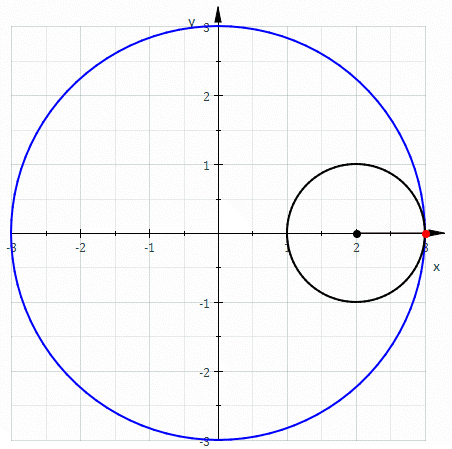demography: The study of human populations using statistical concepts and techniques.

De Moivre’s theorem: A theorem which states the relationship between complex numbers and their exponentiation through trigonometry.De Morgan's laws: Statements relating the union and intersection of sets with their negations. Or more generally, relating the AND and OR operators in logic with the negations of the propositions/sentences.

(A B)' = A' U B'

(A U B)' = A' U B'

denary: Adjective describing the property of base 10, also decimal.

denial of the antecedent: A logical fallacy that (falsely) claims a statement and its converse to be (generally) logically equivalent.

denominate number: The numerical part of a physical quantity. As opposed to the units, which is the denomination.

denomination: A particular unit within a set of units of the same type. (e.g. CM within the set of units for lengths.)

denominator: The part of the fraction represented as under (and to the right, in the case where a solidus is used.) the fraction line - the divisor component of the fraction.

density: The measure of mass per volume and related concepts where either constituent measures may be substituted. (e.g. energy density, charge density.)

denumerable: Can be enumerated, countable.

dependent equations: 1. A system of equations whose set of solutions is a subset of another system of equations. The former set of equations is then dependent on the latter. If the two sets coincide (thus subset, but not proper subsets, of each other), then the sets of equations are interdependent.

2. A system of equations where it is possible to partition the equations such that one subset is dependent (in the sense of 1) to another (non-intersecting) subset.

dependent variable: Also known as a response variable, an explained variable, an outcome variable. As opposed to an independent variable.

depression, angle of: The measure of a plane angle from the horizontal towards the downwards direction.

derivative: The derivative is the function representing the rate of change of the value of an original function with respect to the a variable, also known as a derived function. A classical definition of derivatives define it as the limit (function) of the sequence of functions which represents the increment in the value for a fixed increase of the argument.In physics, mechanics and engineering, derivatives are commonly taken with respect to time: such as velocity and accelration.

derived equation: An equation that results from applying known techniques (augmenting both sides of an equation in the same way, substitution of one equation into another etc.).

derived set: The set of all limit points of a subset of a topological space.

Desargues's theorem: A theorem in geometry which states for two triangles where the lines joining corresponding vertices intersect at the same point, the (infinite) lines which coincides with corresponding sides intersect such that the three points of intersections lie on the same straight line.Descartes's rule of signs: A theorem which states that the number of positive roots of a real polynomial has the same parity as and is less than the number of sign changes of coefficients between consecutive non-zero terms when ordered by degree.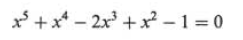In the above polynomial, there are three changes of sign (as the x term is zero), so that the number of positive roots is odd (since 3 is also odd) and is 3 or less. (i.e. 1 or 3 positive roots.)

descriptive statistics: As opposed to inferential statistics, descriptive statistics aim to summarise a set of data in a process where some information (perhaps deemed neglible or irrelevant) is inherently lost, while what is considered important or significant is retained.

designed experiment: The collection of data planned and executed with specific methodolgies so that desirable characteristics remain.

det: A shorthand for the determinant operator.

determinant: A scalar quantity associated with a square matrix, or the transformation represented by the square matrix is a certain coordinate system. Often written as |A| or det A for a matrix A, it is calculated from the elements of the matrix.

For a 2x2 matrix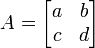the determinant can be calculated by ad – bc.

deterministic model: A mathematical model where the states of a model is determined completely by parameters of the model as well as prior states of the model only.

developable: The property of a surface where the surface can be transformed into a plane figure such that angles and distances remain the same.

deviance: A measure of how much a data set fits a statistical model.

deviation: The difference between an observation and a reference value.

d.f.: A shorthand for degrees of freedom.

diagonal: 1. A line segment between two vertices of a polytope which are not joined by an edge.

2. Related to definition 1, collection of mathematical objects which is conceptually, graphically or representation-wise resembles the analoguous concept of the collect of points forming a line segment. (e.g. a collection of entries of a matrix as in the main diagonal or a collection of digits in a list of numbers as in the diagonal argument.)

diagonalizable matrix: A square matrix M such that P-1MP is a diagonal matrix for some invertible matrix P.

diagonal matrix: A square matrix whose only non-zero entries are on the main diagonal.

diagonal point: The three points of intersections for (three) pairs of lines which (each) contain 2 (distinct) vertices of the quadrilateral.

diametrically opposed: The relationship between two endpoints of a line segment which is the diameter. Also known as antipodal points.

diameter: A line segment containing the centre with both ends on the circumference of a circle.

dichotomous data: Data where all entries fall into exactly one of two categories.

dichotomous variable: A variable where each elements in the population must have exactly one of the two possible values.

dichotomy: The partitioning of a set into two subsets where every elements belongs to exactly one of the two subsets, in other words, the two subsets are collectively exhaustive and mutually exclusive.

difference: The value resulting from a subtraction.

difference equation: A discrete analogue of differential equations which states the general relationship amongst differences between terms of relative positions in a sequence.

differentiable: The property of having a derivative. A real function of one variable is differentiable at a point a if the following limit exists:A function is differentiable over an interval if the function is differentiable at all points within the interval and a function is differentiable if it is differntiable at all points.

differential calculus: One of two main parts of calculus which is concerned with the study of derivatives, as opposed to integral calculus.

differential coefficient: Another class="d-title" name for a derived function or a derivative.

differential equations: A continuous analogue of difference equations. It states the general relationship between derivatives and the variables involved.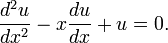The example above is a second degree (second derivative) linear (none of the derivatives are raised to a higher power) ordinary (no partial derivatives) differential equation.

differential geometry: The study of geometric objects with techniques of calculus.

differential operator: A symbolic representation of an operation involving differentiation (either ordinary or partial) of (all of or parts of) the operand, which is a function.

differentiation: The process of determining the derivative (also known as derived function) of a function.

digit: Symbols which are the components in a positional number system.

digital root: The value of an integer modulo 9. The class="d-title" name is used mosting in its use in the verification system called casting out nines.

digraph: A directed graph in graph theory, where each edge of a graph is associated with a direction - i.e. one of the two vertices adjacent to the edge towards which the edge is considered to point.

dihedral angle: The angle between two intersecting planes - also, the same as the angle between the normals of the planes.

Dijkstra's algorithm: An algorithm for finding shortest paths where edges of the graph are all (non-negatively) weighted.

dilation: A transformation where a figure is stretched.

dim: A shorthand or an operator for counting the dimensions of a space.

dimension: Several related concepts informally revolving around the idea of the number of indices needed to describe all elements of a mathematical object where "adjacent" elements receive "adjacent" numberings.

dimensional analysis: A procedure for verifying a formula by the fact that physical laws remain the same regardless of the units used for the physical quantities involved. Dimensional analysis does not check for the value (and placement) of constants acting as coefficients, however.

Diophantine analysis: An area of mathematics concerned with Diophantine equations.

Diophantine equation: Polynomials of more than one variable which has the additional constraint that each variable is allowed to be integers only, in the solution.

directed angle: An angle with a sense of direction from some reference orientation and originating line. A common example is the use of signed angles to denote the angle as well as the sense, clockwise or anti-clockwise, from the intial line in a polar coordinate system.

directed graph: Also known as a digraph.

directed line: A line together with a sense of direction which must be one of the two parallel to the line.

directed number Also known as a signed number, a quantity which admits both positive and negative values.

direction angles: The direction of a vector (line) as represented by the angle it makes with the positive direction of the coordinate axes in a cartesina coordinate system.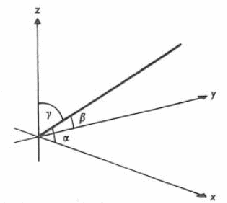direction cosines: The cosine values of the direction angles used for the same purposes.

direction ratios: The ratio of the direction angles, representing the direction of a vector with 2 numbers as opposed to 3 in the cases of the direction angles or the direction cosines.

direct iteration: The iterative method for finding the solution of an equation by rearranging the equation.

directly congruent: Two shapes where either can be made to coincide with the other with a combination of translations and rotations. i.e. congruence with the same orientation.

directly proportional: The property between two variable quantities such that the ratio of the quantities remain constant. (As opposed to partial proportions.)

direct proof: A proof of a statement which does not first prove its contrapositive, or disprove its negation.

directrices of an ellipse: A pair of parallel lines also parallel to the minor axis (thus perpendicular to the major axis) one of which can be used, together with the corresponding (closer) focus, to define the ellipse in question.

directrices of a hyperbola: Two parallel lines, one of which, together with one of the foci, defines the hyperbola through a ratio of the distances to each, known as the eccentricity.

directrix: A line which, together with a point called the focus, defines the set of points which form a conic section.

directrix of a parabola: A line, not intersecting the parabola and perpendicular to the axis of symmetry, which, together with the focus, defines the parabola in question.

direct trigonometric function: Trigonometric functions whose values (range) are the trigonometric ratios. (i.e. sine, cosine, tangent, secant, cosecant and cotangent; NOT arcsine, arccosine, arctangent, arcsecant, arccosecant and arccotangent whose arguments (domains) are the trigonometric ratios.)

Dirichlet's test: A condition for the convergence of a series where, the combination of corresponding terms from a monotonicall`y decreasing real sequence an and a complex sequence bn whose sequence of partial sums is bounded, produces a convergent seriesDirichlet's theorem: A theorem on arithmetic sequences which states that there are infinitely many prime numbers in any arithmetic sequence whose first term and common difference are coprime.

disc: The set of points on a plane which are within a specified distance from a specified point on the plane.

disk: See disc.

disk method: A way of finding the volume of revolution of a solid by considering the volume as the limit of the sums of progressively thinner discs.

disconnected graph: A graph (in the graph theory sense) where there exist two vertices such that there are no paths from one vertex to the other.

discontinuity: A point in the domain of a function at which the function is discontinuous. There are several different types of discontinuity, defined as follows:

1. jump discontinuity - both the left-hand and right-hand limits exist for a point but they are of different values

2. Removable discontinuity - both the left-hand and right-hand limits exist for a point, they have the same value but they do not match the value of the function at the point or the value is not defined at the point.

3. infinite/essential discontinuity - either one or both of the left-hand and right-hand limits do not exist.

discontinuous function: A function which contains a discontinuity.

discrete distribution: A function which describes the probability of a discrete random variable taking its various values.

discrete random variable: A random variable which can only take countably many values (excluding those with probability zero)..

discrete set: Informally, a set is discrete if all members have space between it and any other member of the set (where there "could have" members which belongs to the set but doesn't).

discrete variable: A variable that takes values from a finite or countable set, such as the number of legs of an animal

discriminant: The sum of the squares of differences between all roots of a polynomial. In the case of a quadratic ax2 + bx + c (polynomial of order 2), the discriminant is (x1-x2)2 = b2 - 4ac.

disjoint: A collection of sets are disjoint if their pairwise intersections are all empty.

disjunctive syllogism: Argument of the form, "if A or B, then not A implies B" where the 'or' is an exclusive or.

dispersion: The variability of a quantity. In this sense, a data set can be seen as multiple instances of a variable..

dissection: The division of a set into collectively exhaustive but mutually exclusive sets, also known as a partition.

distance: The length of the shortest line segment connecting elements of one object with another.

distribution: 1. A function which describes the probability of a random variable taking various values.

2. The generalised concept of a function.

distributive: The property of an operation on another where (for each element) the order of the operations are comutative.

div: A common shorthand for the differential operator divergence.

divergence: A differential operator on a vector function such that, for a vector F = Ui + Vj + Wk in the Cartesian coordinate system,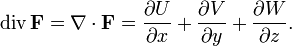divergent integral: An improper integral (infinite integral) with no definite/obvious limit.

divergent product: An infinite product without a non-zero finite limit.

divergent sequence: A sequence whose terms do not tend towards a finite value.

divergent series: A series whose sequence of partial sums is divergent. Note that the terms of a convergent sequence can form a divergent series, with the harmonic sequence being a prime exmaple:divide: To calculate division.

divisible: 1. The relationship between two integers where the quotient of one dividing by another is another integer.

2. The relationship between two polynomials where the quotient of one dividing by another is another polynomial.

division: The inverse operation of multiplication. Note that while multiplication is repeated addition, division is not repeated subtraction.

division sign: The symbol ÷ which resembles a fraction (with the dots representing the numerator and denominator).

divisor: Also known as a factor, it is a number which can divide another number such that the quotient is an integer.

dodecagon: A polygon with twelve sides.

dodecahedron: Any polyhedron with twelve faces.

d.o.f.: An abbreviation for degrees of freedom, like d.f..

domain: The set of values as arguments for a function for which the values are defined.

dot product: Another class="d-title" name for the scalar product of 2 vectors, due to the usual way of distinguishing this type of multiplication from another type (the cross product or vector product) by using a dot.

double-angle formulae: A number of trigonometric identites that relates, for a particular trigonometric function , the value of an argument with the value of the same function with double the argument.

double cone: A geometric figure consisting of two right circular infinite cones placed such that they intersect at only the apex and they share the same axis of rotational symmetry.double cusp: See cusp.

double integral: An integral of an integral with respect to two different variables.

double root: A root of a polynomial which can be thought of as the overlap of 2 roots, either through studying solutions of "neighbouring" polynomials with slightly different coefficients, or through the complete factorisation of the polynomial. A double root is represented on the graph of the function by a skim across/bounce on the x-axis.

double tangent: A tangent that makes contact with and is parallel to a curve at two distinct points..

doubling time: The time for a quantity to double where the quantity is in exponential growth. The opposite idea to half-life.

drag: A force resisting relative velocity of an object within a fluid.

dual game: A game where the optimal strategy for each player is to cooperate, as opposed to a zero-sum game.

duality: A number of related concepts revolving around the idea that the structure of statements remain true for certain commutation (juxtaposition) of the representation of mathematical objects within such statements.

dual theorem, elements, etc.: New theorems produced by apply the concept of duality on to existing theorems, the corresponding elements of a mathematical object within the concept of duality etc.

dummy activity: An edge of zero weight added to a digraph to simplify the algorithms in critical path analysis.

dummy variable: 1. a bound variable

2. A indicator function, taking either a value of 1 or 0, which indicates and takes into account in calculations, the truth value of the presence of a factor which is considered to be significant in the model.

duodecimal notation: The visual representation of a base 12 positional number system (called the duodecimal system) where two additional digits are used to represent ten and eleven - usually A abd B respectively.

duodecimal system: A number system which expresses numbers in terms of powers of 12.

duplication of the cube: Ancient, classical mathematical problem belonging to the same category as trisecting an angle and squaring a circle - all of these are supposed to be solved by straight edge and compasses, and all of these have since been proven to be impossible.

dynamics: The study of motion, its changes and its cause of changes (i.e. forces) within the branch of mechanics.

dyne: A non-SI unit of force.

A   B   C   D   E   F   G   H   I   J   K   L   M   N   O   P   Q   R   S   T   U   V   W   X   Y   Z

Genius is one percent inspiration and ninety-nine percent perspiration.